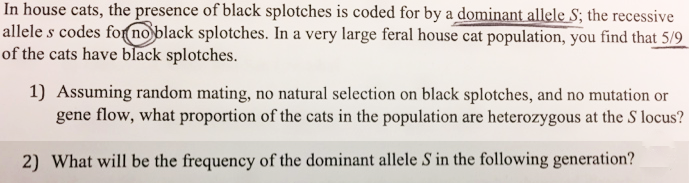# Problem: In house cats, the presence of black splotches is coded for by a dominant allele S; the recessive allele s codes for no black splotches. In a very large feral house cat population, you find that 5/9 of the cats have black splotches.A. Assuming random mating, no natural selection on black splotches, and no mutation or gene flow, what proportion of the cats in the population are heterozygous at the S locus?B. What will be the frequency of the dominant allele S in the following generation?

###### FREE Expert Solution

The relative frequency of cats with homozygous recessive trait, ss, is 0.44.

Assuming the sample follows Hardy-Weinberg equilibrium, p2 + 2pq + q2 = 1, the value for qis 0.44. The value of q, therefore, is 0.67 (the square root of 0.44). And since p + q = 1, the value of p would be 0.33.###### Problem Details

In house cats, the presence of black splotches is coded for by a dominant allele S; the recessive allele s codes for no black splotches. In a very large feral house cat population, you find that 5/9 of the cats have black splotches.

A. Assuming random mating, no natural selection on black splotches, and no mutation or gene flow, what proportion of the cats in the population are heterozygous at the S locus?

B. What will be the frequency of the dominant allele S in the following generation?